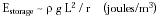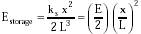Nanomedicine, Volume I: Basic Capabilities

Robert A. Freitas Jr., Nanomedicine, Volume I: Basic Capabilities, Landes Bioscience, Georgetown, TX, 1999

6.2.2 Mechanical Energy Storage

6.2.2.1 Pendulums and Springs

Energy may also be stored in mechanical systems. A gravitational pendulum of cord length r with a bob of density r and characteristic diameter L that is tangentially displaced a distance Dx has potential energy ~r L3 g stored in a volume of order ~r L Dx for small Dx, hence energy density is approximately:{Eqn. 6.2}

Taking r = 2000 kg/m3, g = 9.81 m/sec2 and L = r = 1 micron, then Estorage = 2 x 102 joules/m3, the same as Eqn. 6.1, as expected.

Stretched springs provide significantly greater energy storage capacity. For example, a diamondoid spring of size ~L and stretching stiffness ks has harmonic potential (1/2) ks x2 for a displacement x and volume L3, with energy density:{Eqn. 6.3}

where (x/L) = strain and E = Young's modulus. Conservatively taking strain = 5% and E = 1.05 x 1012 N/m2 for diamond, then Estorage = 1.3 x 109 joules/m3. However, strains may be applied in three dimensions as well as in tension, shear, or torsion, so total energy storage may be somewhat higher. The fracture surface energy of the weakest {111} diamond plane Ef = 5.3 joules/m2, and the distance between {111} planes is Lplane ~ 0.24 nm given that there are ~1.8 x 1019 bonds/m2,10 so the theoretical maximum mechanical energy storage density in a diamond block is Estorage ~ Ef / Lplane = 2 x 1010 joules/m3.

Last updated on 18 February 2003